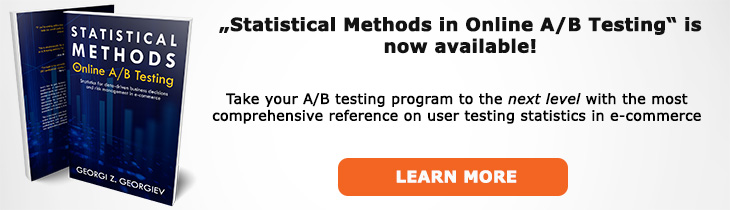# What does "Null Hypothesis Statistical Test" mean?

Definition of Null Hypothesis Statistical Test in the context of A/B testing (online controlled experiments).

## What is a Null Hypothesis Statistical Test?

Aliases: NHST

A Null-Hypothesis Statistical Test (NHST, sometimes Null Hypothesis Significance Test), is a statistical procedure in which a null hypothesis is posed, data related to it is generated and the level of discordance of the outcome with the null hypothesis is assessed using a statistical estimate. The statistical test is most often a Z-Test, T-test or an appropriate equivalent. It's outcome is usually communicated in terms of an observed significance level, a.k.a. p-value which describes how unexpected (unusual, surprising) the actual outcome is, thus shifting the burden of evidence towards any proponents of the null hypothesis just to that extent.

Formally the p-value is expressed as a function of the data x0: p(x0) = P(d(X) > d(x0); H0). This expression makes it obvious that as a tool for causal inference the role of the p-value is to be plugged either in a modus tollens or an equivalent argument from coincidence. In a strict Null-Hypothesis Statistical Test one can either reject the null hypothesis or to fail to reject it, although most practitioners follow an interpretation which introduces an alternative hypothesis and a logic that permits the acceptance of the null hypothesis, to an extent, and the p-value alone is not enough for this to be warranted.

Despite the many criticism over the years the core logic and capacities of an NHST remain key in making data speak in the presence of uncertainty. A properly conducted NHST-based A/B test is the gold standard when a conversion rate optimization specialist needs to provide data to back up a point or evidence to expose an untenable position.

## Related A/B Testing terms

Null Hypothesis

Hypothesis

Significance Test

Hypothesis Testing

## Articles on Null Hypothesis Statistical Test

Like this glossary entry? For an in-depth and comprehensive reading on A/B testing stats, check out the book "Statistical Methods in Online A/B Testing" by the author of this glossary, Georgi Georgiev.## Glossary Index by Letter

Select a letter to see all A/B testing terms starting with that letter or visit the Glossary homepage to see all.